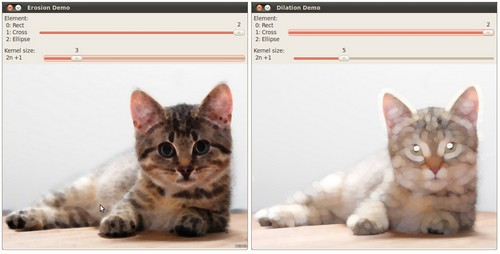OpenCV  4.6.0 Open Source Computer Vision
Eroding and Dilating

Prev Tutorial: Smoothing Images

Next Tutorial: More Morphology Transformations

Original author Ana Huamán
Compatibility OpenCV >= 3.0

## Goal

In this tutorial you will learn how to:

• Apply two very common morphological operators: Erosion and Dilation. For this purpose, you will use the following OpenCV functions:
Note
The explanation below belongs to the book Learning OpenCV by Bradski and Kaehler.

## Morphological Operations

• In short: A set of operations that process images based on shapes. Morphological operations apply a structuring element to an input image and generate an output image.
• The most basic morphological operations are: Erosion and Dilation. They have a wide array of uses, i.e. :
• Removing noise
• Isolation of individual elements and joining disparate elements in an image.
• Finding of intensity bumps or holes in an image
• We will explain dilation and erosion briefly, using the following image as an example:### Dilation

• This operations consists of convolving an image $$A$$ with some kernel ( $$B$$), which can have any shape or size, usually a square or circle.
• The kernel $$B$$ has a defined anchor point, usually being the center of the kernel.
• As the kernel $$B$$ is scanned over the image, we compute the maximal pixel value overlapped by $$B$$ and replace the image pixel in the anchor point position with that maximal value. As you can deduce, this maximizing operation causes bright regions within an image to "grow" (therefore the name dilation).
• The dilatation operation is: $$\texttt{dst} (x,y) = \max _{(x',y'): \, \texttt{element} (x',y') \ne0 } \texttt{src} (x+x',y+y')$$
• Take the above image as an example. Applying dilation we can get:• The bright area of the letter dilates around the black regions of the background.

### Erosion

• This operation is the sister of dilation. It computes a local minimum over the area of given kernel.
• As the kernel $$B$$ is scanned over the image, we compute the minimal pixel value overlapped by $$B$$ and replace the image pixel under the anchor point with that minimal value.
• The erosion operation is: $$\texttt{dst} (x,y) = \min _{(x',y'): \, \texttt{element} (x',y') \ne0 } \texttt{src} (x+x',y+y')$$
• Analagously to the example for dilation, we can apply the erosion operator to the original image (shown above). You can see in the result below that the bright areas of the image get thinner, whereas the dark zones gets bigger.## Explanation

Note
Additionally, there are further parameters that allow you to perform multiple erosions/dilations (iterations) at once and also set the border type and value. However, We haven't used those in this simple tutorial. You can check out the reference for more details.

## Results

Compile the code above and execute it (or run the script if using python) with an image as argument. If you do not provide an image as argument the default sample image (LinuxLogo.jpg) will be used.

For instance, using this image:We get the results below. Varying the indices in the Trackbars give different output images, naturally. Try them out! You can even try to add a third Trackbar to control the number of iterations.(depending on the programming language the output might vary a little or be only 1 window)